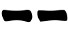# Four Coins

Four coins can be used to easily generate hexagram lines with yarrow stalks probabilities.

To my knowledge this method has been devised by Stuart Anderson (the orginal site is no longer online)  and proceeds as follows:

1. Pick three identical coins and one coin that is notably different from the others
2. Throw the four coins;
3. Look at the single different coin:
• If it's tail, draw;
• If it's head, draw;
4. Look at the four coins, if among them there are exactly three head, it is a moving line.
5. Repeat steps 1-4 drawing hexagram lines from bottom to top.

#### Probabilities

The table below summarizes the possible outcomes of this metods, the results that generate a moving line are highlighted in red.

By counting, it's easy to see that the probabilities are:
Prob(6) = 1/16
Prob(8) = 7/16
Prob(7) = 5/16
Prob(9) = 3/16
Prob(yin) = Prob(yang) = 1/2

1.2.